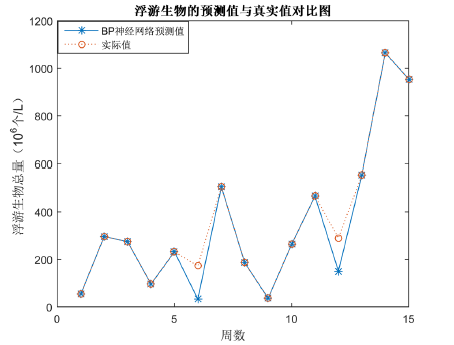﻿ 基于主成分分析的BP神经网络在水华预测中的应用

# 基于主成分分析的BP神经网络在水华预测中的应用Application of BP Neural Network Based on Principal Component Analysis in Algal Bloom Prediction

Abstract: With the intensification of water pollution and eutrophication of freshwater ecosystems, large areas of algal bloom have been included, which not only destroy the ecosystems, but also cause huge economic losses. Therefore, it is very important to predict the occurrence of algal bloom according to the physical and chemical factors of water body. Firstly, according to the data of the pond for 1~15 weeks, the main influencing factors of 13 physical and chemical factors affecting the total plankton were analyzed based on principal component analysis (PCA). The main influencing factors of algal blooms were total nitrogen, transparency, dissolved oxygen, ammonium nitrogen, salinity, total phosphorus and dissolved oxygen. Secondly, according to the main seven physical and chemical factors identified as the input layer of BP neural network, the plankton biomass was used as the output layer to predict the occurrence of algal bloom. The results show that the fitting coefficient between the predicted result and the true value of the BP neural network model based on principal component analysis is as high as 0.9912. Therefore, the research method in this paper can effectively predict the occurrence of algal bloom.

1. 引言

2. 研究方法

2.1. 主成分分析法

1) 基本思想

$X={\left({x}_{i,j}\right)}_{i=1,2,\cdots ,m,\text{\hspace{0.17em}}j=1,2,\cdots ,m}$ . (1)

${Z}_{k}={c}_{k1}{X}_{1}+{c}_{k2}{X}_{2}+\cdots +{c}_{kp}{X}_{p},\text{\hspace{0.17em}}k=1,2,\cdots ,m$ . (2)

2) 分析过程

① 计算相关系数矩阵 $R={\left({r}_{ij}\right)}_{p×p}$ ，其中

${r}_{ij}=\frac{\underset{k=1}{\overset{n}{\sum }}\left({x}_{ki}-{\stackrel{¯}{x}}_{i}\right)\left({x}_{kj}-{\stackrel{¯}{x}}_{j}\right)}{\sqrt{\underset{k=1}{\overset{n}{\sum }}{\left({x}_{ki}-{\stackrel{¯}{x}}_{i}\right)}^{2}{\left({x}_{kj}-{\stackrel{¯}{x}}_{j}\right)}^{2}}}$ . (3)

② 计算特征值与特征向量

③ 计算主成份贡献率 ${z}_{i}$ 及累计贡献率 ${Z}_{i}$

${z}_{i}={\lambda }_{i}/\underset{k=1}{\overset{p}{\sum }}{\lambda }_{k},\text{\hspace{0.17em}}i=1,2,\cdots ,p$ . (4)

${Z}_{i}=\underset{k=1}{\overset{p}{\sum }}\left({\lambda }_{k}/\underset{k=1}{\overset{p}{\sum }}{\lambda }_{k}\right),\text{\hspace{0.17em}}i=1,2,\cdots ,p$ . (5)

④ 计算主成份载荷 ${l}_{ij}$

${l}_{ij}=p\left({z}_{i},{x}_{j}\right)=\sqrt{{\lambda }_{j}-{e}_{ij}},\text{\hspace{0.17em}}\text{\hspace{0.17em}}i,j=1,2,\cdots ,p$ . (6)

2.2. BP神经网络模型

BP算法具有梯度性，也称为快速下降法，其迭代基本思想是：从一个初始点 ${w}_{0}$ 出发，计算在点 ${w}_{0}$Figure 1. BP neural network topology

BP神经网络具体算法如下：

1) 初始化网络及学习参数。为加快网络学习将隐含层和输出层各节点的连接权值、神经元阈值规范化处理，其表达式如下：

$\left\{\begin{array}{l}{x}_{k}^{new}=\frac{0.002+0.996\left({x}_{k}^{old}-\mathrm{min}{x}_{k}^{old}\right)}{\mathrm{max}{x}_{k}^{old}-\mathrm{min}{x}_{k}^{old}}\\ {y}_{k}^{new}=\frac{0.002+0.996\left({y}_{k}^{old}-\mathrm{min}{y}_{k}^{old}\right)}{\mathrm{max}{y}_{k}^{old}-\mathrm{min}{y}_{k}^{old}}\end{array}$ , (7)

2) 利用数据对网络进行训练，计算网络的输入、输出值。

${s}_{j}^{k}=\underset{i=1}{\overset{n}{\sum }}{a}_{i}^{k}{w}_{ij}-{\theta }_{j},\text{\hspace{0.17em}}\text{\hspace{0.17em}}\text{\hspace{0.17em}}{a}_{j}^{k}=\frac{1}{1+{\text{e}}^{-{s}_{j}^{k}}},\text{\hspace{0.17em}}\text{\hspace{0.17em}}j=1,2,\cdots ,p$ . (8)

${l}_{t}^{k}=\underset{i=1}{\overset{p}{\sum }}{b}_{t}^{k}{v}_{t},\text{\hspace{0.17em}}\text{\hspace{0.17em}}{b}_{t}^{k}=\frac{1}{1+{\text{e}}^{-{l}_{t}^{k}}},\text{\hspace{0.17em}}\text{\hspace{0.17em}}t=1,2,\cdots ,q$ . (9)

3) 误差逆传播，利用梯度下降法对各层连接层及阈值进行调整。

${E}_{k}=\underset{t=1}{\overset{q}{\sum }}\frac{{\left({y}_{k}^{k}-{c}_{t}^{k}\right)}^{2}}{2}$ , (10)

4) 修正权值与阈值。

5) 若网络的全局误差小于指定的值，则算法转入第6步，否则转入第2步。

6) 计算输出层。

7) 计算网络训练误差。

BP神经网络仿真测试结束后，通过计算真实值与输出值的偏差情况，对网络训练的泛化能力进行评价，选取决定系数R2评价模型的性能，其中

${R}^{2}=\frac{{\left(l\underset{i=0}{\overset{l}{\sum }}{\stackrel{^}{y}}_{i}{y}_{i}-\underset{i=0}{\overset{l}{\sum }}{\stackrel{^}{y}}_{i}\underset{i=0}{\overset{l}{\sum }}{y}_{i}\right)}^{2}}{\left(l\underset{i=0}{\overset{l}{\sum }}{\stackrel{^}{y}}_{i}^{2}-{\left(\underset{i=0}{\overset{l}{\sum }}{\stackrel{^}{y}}_{i}^{2}\right)}^{2}\right)\left(l\underset{i=0}{\overset{l}{\sum }}{y}_{i}^{2}-{\left(\underset{i=0}{\overset{l}{\sum }}{y}_{i}^{2}\right)}^{2}\right)}$ , (11)

3. 实例分析

3.1. 池塘水华主要理化因子Table 1. Principal component solution data sheetTable 2. The main physical and chemical factors of water bloom and biological data sheet

3.2. 结果与分析

1) 模型的输入、输出参数的选择

2) 模型的结构设计

3) 模型网络参数的选取及参数设定

4) 模型检验与仿真分析Figure 2. Planktonic predicted values and real value comparison chartFigure 3. Neural network fitting coefficient map

4. 结论

1) 通过主成分分析可得影响水华发生的主要理化因子，包括总氮、透明度、溶解氧(COD)、铵态氮、盐度、总磷。这7个理化因子的累积贡献率为96.5177%。

2) 所构建的神经网络模型，由于神经网络模型具有较强的非线性映射能力，经过训练后的预测值与真实值吻合度较高。

3) 基于主成分分析的BP神经网络降低了网络输入的层数，提高了程序运行效率，从而提高了神经网络的性能，对水华预测有较好的效果。

 窦明, 谢平, 夏军, 沈晓鲤, 方芳. 汉江水华问题研究[J]. 水科学进展, 2002, 13(5): 557-561

 陈云峰, 殷福才, 陆根法. 水华爆发的突变模型——以巢湖为例[J]. 生态学报, 2006, 26(3): 878-883.

 杜桂森, 王建厅, 张为华, 冯伶亲, 刘静. 官厅水库水体营养状况分析[J]. 湖泊科学, 2004, 16(3): 277-281.

 全为民, 严力蛟, 虞左明, 焦荔. 湖泊富营养化模型研究进展[J]. 生物多样性, 2001, 9(2): 168-175.

 郝启文, 王小艺, 许继平, 刘载文, 盛璐, 何多多. 湖库水质监测与水华预警信息系统[J]. 计算机工程, 2013, 39(1): 287-289+293.

 孔繁翔, 马荣华, 高俊峰, 吴晓东. 太湖蓝藻水华的预防、预测和预警的理论与实践[J]. 湖泊科学, 2009, 21(3): 314-328.

 仝玉华, 周洪亮, 黄浙丰, 张宏建. 一种自优化RBF神经网络的叶绿素a浓度时序预测模型[J]. 生态学报, 2011, 31(22): 6788-6795.

 姚俊杨, 许继平, 王小艺, 黄振芳. 基于深度学习的湖库藻类水华预测研究[J]. 计算机与应用学, 2015, 32(10): 1265-1268.

 李大刚, 王小艺, 刘载文, 许继平, 赵星, 戴军. 过程神经网络水华预测方法研究[J]. 计算机与应用化学, 2011, 28(2): 173-176.

 桑慧茹, 王丽学, 陈韶明, 孙娟, 李司瑾. 基于主成分分析的RBF神经网络在需水预测中的应用[J]. 水电能源科学, 2017, 35(7): 58-61.

 苏键, 陈军, 何洁. 主成分分析法及其应用[J]. 轻工科技, 2012, 28(9): 12-13+16.

 万金保, 曾海燕, 朱邦辉. 主成分分析法在乐安河水质评价中的应用[J]. 中国给水排水, 2009, 25(16): 104-108.

 任黎, 董增川, 李少华. 人工神经网络模型在太湖富营养化评价中的应用[J]. 河海大学学报(自然科学版), 2004, 32(2): 147-150.

Top